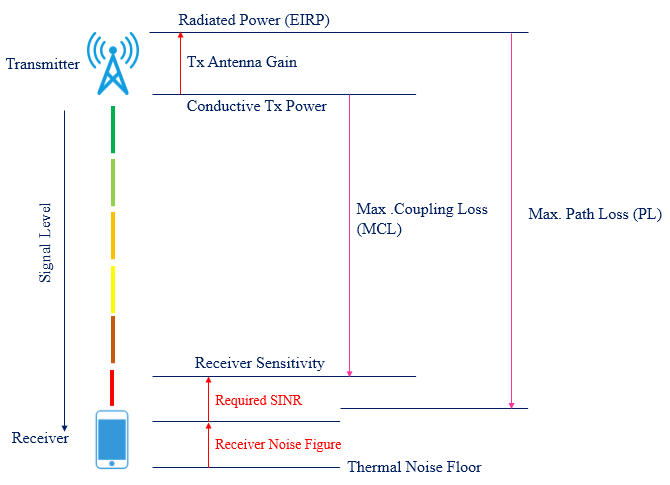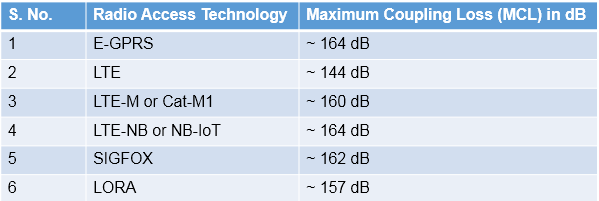# Maximum Coupling Loss (MCL) and Maximum Path Loss (MPL)

Maximum Coupling Loss has been chosen by 3GPP as the metric to evaluate coverage of a radio access technology. In theory, it can be defined as the maximum loss in the conducted power level that a system can tolerate and still be operational (defined by a minimum acceptable received power level). MCL can be calculated as the difference between the conducted power levels measured at the transmitting and receiving antenna ports as the reference point, the directional gain of the antenna is not considered when calculating MCL.Coverage can also be expressed by the Maximum Path Loss (MPL) for a radio technology. Here path loss is the loss in the signal path due to distant propagation, building penetration, etc. of the radiated power. Hence, MPL can be calculated by the difference in radiated power levels at the transmitting and receiving antennas. To determine the MPL also the antenna gain at the transmitter and receiver need to be considered.The difference between MCL and MPL is shown in following figure.•  Noise Figure (NF) + SINR + Noise Floor = Receiver Sensitivity
• Noise Floor = -174 + 10 log10(Bandwidth)
###### Maximum Coupling Loss Supported by Different Radio Access Technologies.Related Posts: